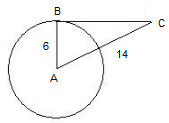# AP Class 10 Maths Chapter 9 Tangents and Secants

The word tangent was introduced by Danish mathematician Thomas Fineke in 1583 and it means to touch in Latin. Let us look at the definition of tangent and secant from AP Class 10 Maths Chapter 9 Tangents and Secants.

• Tangent – It is a line that intersects the circle at one point only
• Secant – It is a line that intersects the circle in two different points

Referring to the AP Board 10th Class Maths Chapter 9 Tangents and Secants notes and solutions are the best way to study for the board exams, understand the concepts, revise the key topics and to ace the Maths paper.

## Tangents of a Circle

• The common point at which the tangent and the circle meet is known as the point of contact.
• The tangent at any point of a circle is perpendicular to the radius through the point of contact.
• The length of tangents drawn from an external point to a circle are equal.
• The area of the segment of a circle can be calculated using the formula
$\frac{x^{\circ}}{360^{\circ}}\times \Pi r^{2}-area\, of \,the \,triangle$

In the next section, let us look at a few solved chapter questions to better understand the concepts discussed in the chapter.

### Class 10 Maths Chapter 9 Tangents and Secants Questions

1. The tangent BC at a point B of a circle of 6 cm meets a line through the centre O at a point C such that AC =14cm. Find the length of BC.Solution:

From the figure, we notice that $AB\perp BC$,

According to Pythagoras theorem,

In $\Delta ABC$,

$AC^2=AB^2+BC^2$

Substituting the values in the equation, we get

$14^2=6^2+BC^2$ $BC^2=14^2-6^2$ $BC^2=160$ $BC=\sqrt{160}=12.65 cm$

The length of BC is 12.65 cm.

Stay tuned to BYJUS to get the latest notification on SSC exam along with AP SSC model papers, exam pattern, marking scheme and more.